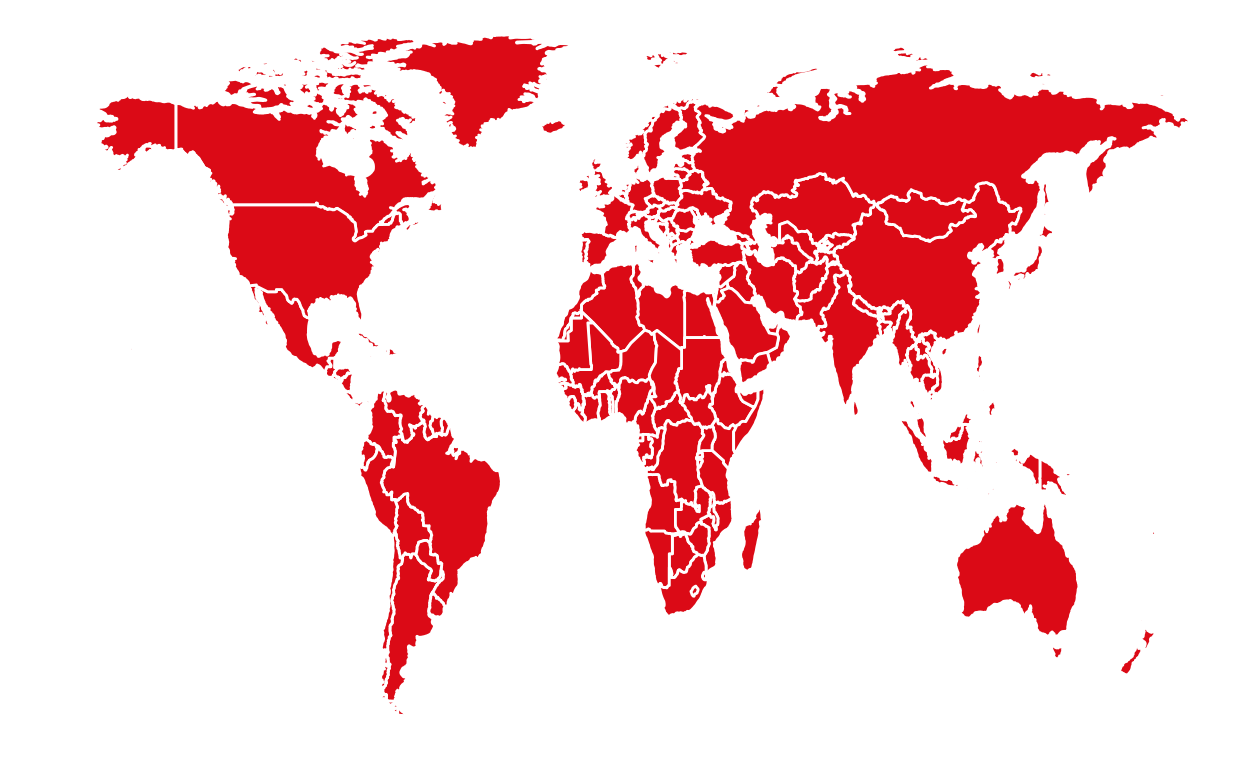# [API] WDI

Access global development data through the WDI API.

01-29-2020## Database description

“The primary World Bank collection of development indicators, compiled from officially-recognized international sources. It presents the most current and accurate global development data available, and includes national, regional and global estimates” (World Development Indicators, The World Bank)

World bank : https://www.worldbank.org/

## Functions

This library gives access to all indicators provided by the World Bank. The functions listed below allow you to search and download specific data from the WDI database.

• WDIsearch()
• WDI()

Each of these functions are detailed in this course and some examples are provided.

### WDIsearch()

The function WDIsearch() takes as an input any string of character and will provide the list of indicators containing this string of character.

For example, we would like to obtain all indicators using the term “GDP” from the database WDI.

``````#Loading the WDI library
library(WDI)

# Search all indicators with the term "GDP"
listOfIndicators <- WDIsearch("GDP")

# List the first 5 indicators
listOfIndicators[1:5,]
``````
``````     indicator
[1,] "5.51.01.10.gdp"
[2,] "6.0.GDP_current"
[3,] "6.0.GDP_growth"
[4,] "6.0.GDP_usd"
[5,] "6.0.GDPpc_constant"
name
[1,] "Per capita GDP growth"
[2,] "GDP (current \$)"
[3,] "GDP growth (annual %)"
[4,] "GDP (constant 2005 \$)"
[5,] "GDP per capita, PPP (constant 2011 international \$) "``````

### WDI()

The function WDI() takes as an input the indicator’s code and the country of the data wanted. It returns the value of the indicator for the countries selected. To search specific dates, it is possible to add as inputs the starting year and the ending year of the data.

For example, it would be interesting to evaluate the total amount of stocks traded in percentage of GDP (CM.MKT.TRAD.GD.ZS) for 4 countries (France - FR; Canada - CA; USA - US; China - CN) from 2000 to 2014. This could be obtained by using the function WDI() with the following inputs:

• country = c(“FR”, “CA”, “US”,“CN”)
• start = 2000
• end = 2014
``````# Access and store data concerning Stocks traded in total value (% of GDP)
stockTraded <- WDI(indicator = "CM.MKT.TRAD.GD.ZS", country = c("FR", "CA", "US","CN"), start = 2000, end = 2016)

``````
``````  iso2c country CM.MKT.TRAD.GD.ZS year

## tl;dr

``````#Loading the WDI library
library(WDI)

# Search all indicators with the term "GDP"
listOfIndicators <- WDIsearch("GDP")

# List the first 5 indicators
listOfIndicators[1:5,]

stockTraded <- WDI(indicator = "CM.MKT.TRAD.GD.ZS", country = c("FR", "CA", "US","CN"), start = 2000, end = 2016)